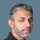cancel
Showing results for
Did you mean:Frequent Visitor

## Countrows les then and more than a value

Hello,

I am trying to create a measure which will count the number of rows in a column that have a number of less than 36 and more than 36 (but not any that are 36).

Would anyone know what is the best way to do this please?

Thank you, Helen

1 ACCEPTED SOLUTIONSuper User

@hellyLoft , Try like

MidYearAdm = CALCULATE(COUNTROWS('Pupil'[week number]),filter(Pupil,Pupil[week number] <36 || Pupil[week number] >36 ))

2 REPLIES 2Super User

@hellyLoft

MidYearAdm = CALCULATE(COUNTROWS('Pupil[week number]),Pupil[week number] <> 36)

________________________

Did I answer your question? Mark this post as a solution, this will help others!.

Click on the Thumbs-Up icon on the right if you like this reply 🙂

Did I answer your question? Mark my post as a solution! and hit thumbs upSuper User

@hellyLoft , Try like

MidYearAdm = CALCULATE(COUNTROWS('Pupil'[week number]),filter(Pupil,Pupil[week number] <36 || Pupil[week number] >36 ))